IF YOU CAN SEE THIS go to /sfa/Nuprl/Shared/Xindentation_hack_doc.html

### Disjoint Union of Two Types

A+B is called the disjoint union of type A with type B.
The members of A+B are gotten by tagging members from A and B. They are inl(a) for a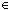A and inr(b) for bB.

inl(a) and inr(b) are sometimes called the left- and right-injections of a and b.

InjCase(xue(u); vf(v))

is the destructor for left- and right-injections.

InjCase(inl(a); ue(u); vf(v)) *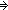e(a)
InjCase(inr(b); ue(u); vf(v)) *f(b)

Thm*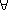z:A+Bz = InjCase(zu. inl(u); v. inr(v))

Some commonly used specializations of InjCase(xue(u); vf(v)) are:

Def isl(x) == InjCase(xy. true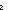z. false)
Def outl(x) == InjCase(xyyz. "???")
Def outr(x) == InjCase(xy. "???"; zz)

Thm*A,B:Type, x:A+B. isl(x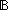Thm*A,B:Type, x:A+B. isl(x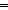outl(xA
Thm*A,B:Type, x:A+B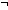isl(xoutr(xB

outl(inl(a)) *a
outr(inr(b)) *b

A+A is essentially the type A with a bit added.

We treat A+B, inl(a), inr(b), and InjCase(xue(u); vf(v)) as primitives, but we could instead have defined them using the pairing operation to add a tag:

Let A+B be isleft: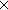if isleft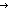A else B fi.

Let inl(a) be <true,a>.

Let inr(b) be <false,b>.

Let InjCase(xue(u); vf(v)) be

x/isleft,z. if islefte(z) else f(z) fi.

(Bywe mean a two-element Boolean type.)

(Sept 2001 - sfa )